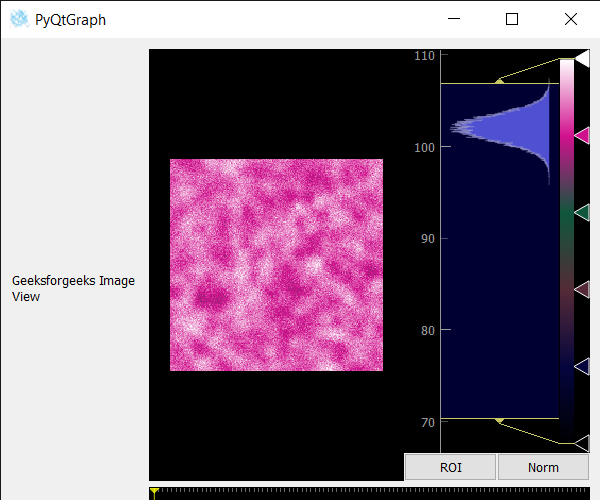# PyQtGraph – Removing Mask of Image View

• Last Updated : 24 Nov, 2021

In this article, we will see how we can remove the mask of the image view object in PyQTGaph. PyQtGraph is a graphics and user interface library for Python that provides functionality commonly required in designing and science applications. Its primary goals are to provide fast, interactive graphics for displaying data (plots, video, etc.). Widget used for display and analysis of image data. Implements many features like displaying 2D and 3D image data. For 3D data, a z-axis slider is displayed allowing the user to select which frame is displayed. Displays histogram of image data with a movable region defining the dark/light levels, editable gradient provides a color lookup table. Mask basically hides the selected part of the image view, the image view will be still there but the user will not be able to see it i.e there will be a mask on it. Only the selected region will be visible other regions will have a mask, it can be set with the help of the setback method.
We can create an image view with the help of the command given below.

```# creating a pyqtgraph image view object
imv = pg.ImageView()```

In order to do this we use clearMask method with the image view object
Argument : It takes no argument
Return : It returns None

Below is the implementation.

## Python3

 `# importing Qt widgets``from` `PyQt5.QtWidgets ``import` `*` `# importing system``import` `sys` `# importing numpy as np``import` `numpy as np` `# importing pyqtgraph as pg``import` `pyqtgraph as pg``from` `PyQt5.QtGui ``import` `*``from` `PyQt5.QtCore ``import` `*`  `# Image View class``class` `ImageView(pg.ImageView):` `    ``# constructor which inherit original``    ``# ImageView``    ``def` `__init__(``self``, ``*``args, ``*``*``kwargs):``        ``pg.ImageView.__init__(``self``, ``*``args, ``*``*``kwargs)`  `class` `Window(QMainWindow):` `    ``def` `__init__(``self``):``        ``super``().__init__()` `        ``# setting title``        ``self``.setWindowTitle(``"PyQtGraph"``)` `        ``# setting geometry``        ``self``.setGeometry(``100``, ``100``, ``600``, ``500``)` `        ``# icon``        ``icon ``=` `QIcon(``"skin.png"``)` `        ``# setting icon to the window``        ``self``.setWindowIcon(icon)` `        ``# calling method``        ``self``.UiComponents()` `        ``# showing all the widgets``        ``self``.show()` `        ``# setting fixed size of window``        ``#self.setFixedSize(QSize(600, 500))` `    ``# method for components``    ``def` `UiComponents(``self``):` `        ``# creating a widget object``        ``widget ``=` `QWidget()` `        ``# creating a label``        ``label ``=` `QLabel(``"Geeksforgeeks Image View"``)` `        ``# setting minimum width``        ``label.setMinimumWidth(``130``)` `        ``# making label do word wrap``        ``label.setWordWrap(``True``)` `        ``# setting configuration options``        ``pg.setConfigOptions(antialias``=``True``)` `        ``# creating image view view object``        ``imv ``=` `ImageView()` `        ``# Create random 3D data set with noisy signals``        ``img ``=` `pg.gaussianFilter(np.random.normal(``            ``size``=``(``200``, ``200``)), (``5``, ``5``)) ``*` `20` `+` `100` `        ``# setting new axis to image``        ``img ``=` `img[np.newaxis, :, :]` `        ``# decay data``        ``decay ``=` `np.exp(``-``np.linspace(``0``, ``0.3``, ``100``))[:, np.newaxis, np.newaxis]` `        ``# random data``        ``data ``=` `np.random.normal(size``=``(``100``, ``200``, ``200``))``        ``data ``+``=` `img ``*` `decay``        ``data ``+``=` `2` `        ``# adding time-varying signal``        ``sig ``=` `np.zeros(data.shape[``0``])``        ``sig[``30``:] ``+``=` `np.exp(``-``np.linspace(``1``, ``10``, ``70``))``        ``sig[``40``:] ``+``=` `np.exp(``-``np.linspace(``1``, ``10``, ``60``))``        ``sig[``70``:] ``+``=` `np.exp(``-``np.linspace(``1``, ``10``, ``30``))` `        ``sig ``=` `sig[:, np.newaxis, np.newaxis] ``*` `3``        ``data[:, ``50``:``60``, ``30``:``40``] ``+``=` `sig` `        ``# setting image to image view``        ``# Displaying the data and assign each frame a time value from 1.0 to 3.0``        ``imv.setImage(data, xvals``=``np.linspace(``1.``, ``3.``, data.shape[``0``]))` `        ``# Set a custom color map``        ``colors ``=` `[``            ``(``0``, ``0``, ``0``),``            ``(``4``, ``5``, ``61``),``            ``(``84``, ``42``, ``55``),``            ``(``15``, ``87``, ``60``),``            ``(``208``, ``17``, ``141``),``            ``(``255``, ``255``, ``255``)``        ``]` `        ``# color map``        ``cmap ``=` `pg.ColorMap(pos``=``np.linspace(``0.0``, ``1.0``, ``6``), color``=``colors)` `        ``# setting color map to the image view``        ``imv.setColorMap(cmap)` `        ``# Creating a grid layout``        ``layout ``=` `QGridLayout()` `        ``# minimum width value of the label``        ``label.setFixedWidth(``130``)` `        ``# setting this layout to the widget``        ``widget.setLayout(layout)` `        ``# adding label in the layout``        ``layout.addWidget(label, ``1``, ``0``)` `        ``# plot window goes on right side, spanning 3 rows``        ``layout.addWidget(imv, ``0``, ``1``, ``3``, ``1``)` `        ``# setting this widget as central widget of the main window``        ``self``.setCentralWidget(widget)` `        ``# QRect object``        ``rect ``=` `QRect(``100``, ``10``, ``300``, ``300``)` `        ``# creating a QRegion``        ``region ``=` `QRegion(rect)` `        ``# setting mask to the image view``        ``imv.setMask(region)` `        ``# removing mask of image view``        ``imv.clearMask()`  `# create pyqt5 app``App ``=` `QApplication(sys.argv)` `# create the instance of our Window``window ``=` `Window()` `# start the app``sys.exit(App.``exec``())`

Output :My Personal Notes arrow_drop_up